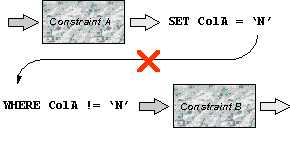Deactivation Between Constraints

The Rules Engine deactivates between constraints at compile time. This happens:

 · When flat files are created.

 · If you run from repository, the first time a particular set of constraints is loaded on the client as a result of row events.

It is used to determine the optimal order of constraints that are handled pre-store.

Example

This illustration shows one of the three situations where the Rules Engine knows that if a constraint A results in a correction, constraint B no longer needs to be considered for the same record. Constraint B is then deactivated by constraint A:On the basis of such dependencies, the constraints that are most likely to deactivate the greatest number of other constraints are evaluated first.

In general, constraint X deactivates constraint Y in the following situations:

 · The post-condition of X and the pre-condition of Y are mutually exclusive. If X succeeds for the current row, Y can no longer be applicable to this row.

 · The post-condition of X and the post-condition of Y are equivalent. If X succeeds from the current row, then there is no point in evaluating Y since the result would be the same.

 · The pre-conditions of X and Y are mutually exclusive. If X applies to the current row, then Y cannot apply to it and vice versa.